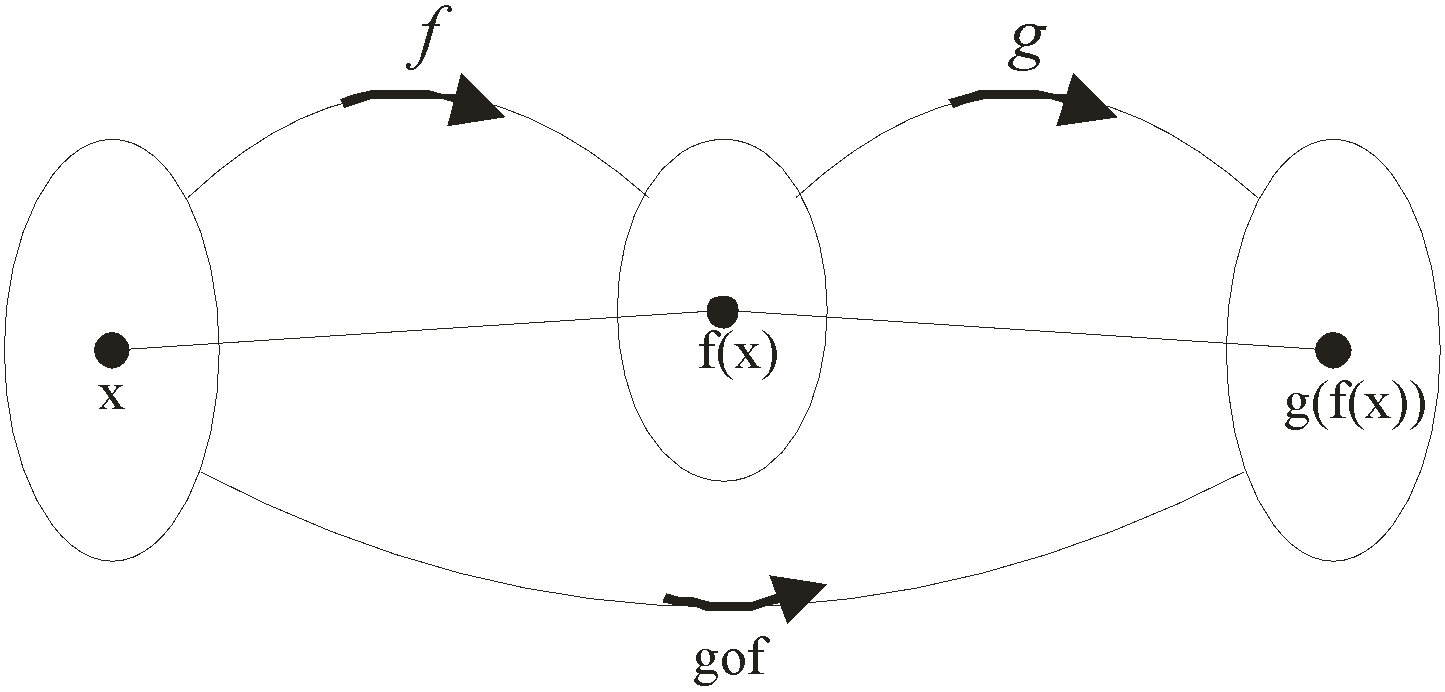BodhiAI
Nov. 11, 2019

#### Composite Function & their Properties:

Composite Functions

Let f :AB and g : BC be two functions. Then a function gof : AC defined by (gof) (x) = g(f(x)), for all xA

is called the composition of f and g.

Let A, B and C be three non-void sets and let f : AB, g: BC be two functions. Since f is a function from A to B, therefore for each xA there exists a unique element f(x)B. Again, since g if a function from B to C, therefore corresponding to f(x)B there exists a unique element g(f(x))C. Thus, for each xA there exists a unique element g(f(x))C.

It follows from the above discussion that f and g when considered together define a new function from A to C.This function is called the composition of f and g and is denoted by gof. We define it formally as follows.Important

a) gof is defined only if for each xA, f(x) is an element of g so that we can take its g-image. Hence for the composition gof to exist, the range of f must be a subset of the domain of g.

b) gof exists iff the range of f is a subset of domain of g. Similarly, fog exists if range of g is a subset of domain of f.

Properties of Composite Functions

P-1 The composition of functions is not commulative

i.e. fog ≠ gof

P-2 The composition of functions is associative

i.e. If f, g, h are any three functions such that (fog) oh and fo(goh) both exist, then

(fog)oh = fo(goh)

P-3 The composition of two bijective function is also a bijective function.

P-4 The composition of any function with the identity function is the function itself.

i.e. If f : A→B, then

foIA = IBof = f